# Equilibrium position

(diff) ← Older revision | Latest revision (diff) | Newer revision → (diff)

of a system of ordinary differential equationsA pointsuch thatis a solution of

(constant in time). The solution itself is also called an equilibrium position. A point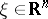is an equilibrium position of

if and only if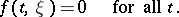Letbe an arbitrary solution of . The change of variablestransforms this solution into the equilibrium position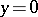of the system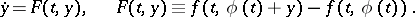Therefore, in stability theory, for example, it is possible to assume, without loss of generality, that the problem always consists of investigating the stability of an equilibrium position at the origin in.

The equilibrium position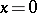of a non-autonomous system

is often called the trivial solution, zero solution, singular point, stationary point, rest point, equilibrium state, or fixed point.

How to Cite This Entry:
Equilibrium position. Encyclopedia of Mathematics. URL: http://encyclopediaofmath.org/index.php?title=Equilibrium_position&oldid=18108
This article was adapted from an original article by N.Kh. Rozov (originator), which appeared in Encyclopedia of Mathematics - ISBN 1402006098. See original article